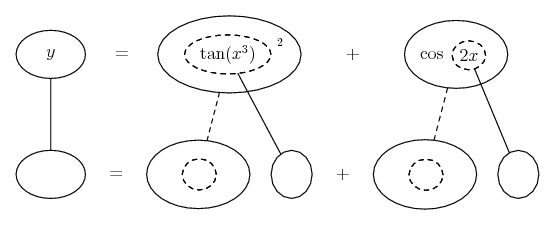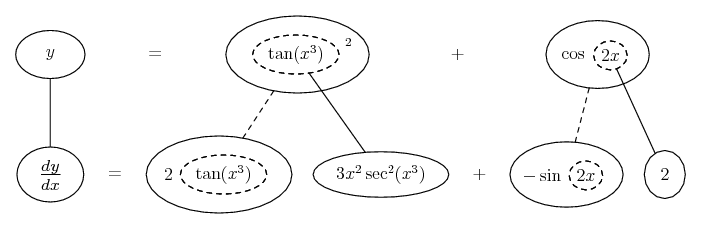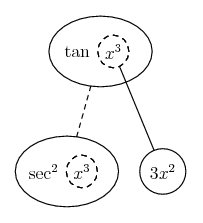1. ## Differenciation

am trying to differentiate these equations
$\displaystyle y = tan^2(x)^3 + cos 2x$ and

$\displaystyle y=(e^x)^2/ln(2x)$

2.Originally Posted by sigma1am trying to differentiate these equations
$\displaystyle y = tan^2(x)^3 + cos 2x$ and

$\displaystyle y=(e^x)^2/ln(2x)$
1) Chain rule.

2) Quotient rule.

3. i know that but am having a bit of problem separating the variable that is making something "U" . i would like to see how it is done if that is not too much of a trouble. am trying to catch up quickly on calculus.

4. U substitution is a topic for integration, not differentiation. If you can show us your work, we will be more than happy to help point you in the right direction.

You may also want to check out this link, which covers product, quotient and chain rules for differentiation: Product Rule, Quotient Rule, and Chain Rule Tutorial

5. Just in case a picture helps...... where... is the chain rule. Straight continuous lines differentiate downwards (integrate up) with respect to x, and the straight dashed line similarly but with respect to the dashed balloon expression (which is the inner function of the composite and hence subject to the chain rule).

Spoiler:... and the inner differentiation zoomed in...________________________________________
Don't integrate - balloontegrate!

Balloon Calculus: Standard Integrals, Derivatives and Methods

Balloon Calculus Drawing with LaTeX and Asymptote!

6.Originally Posted by sigma1am trying to differentiate these equations
$\displaystyle y = tan^2(x)^3 + cos 2x$ and

$\displaystyle y=(e^x)^2/ln(2x)$
1. the derivative of $\displaystyle tan^{2}(x)^3 = 2tan(x^3)sec^2(x^3) 3x^2$

Likewise find out the derivaitve of $\displaystyle cos2x$ and add them up

2. try the quotient rule, show how much effort you put on this problem. Post if you have any errors and they will be fixed.

7.Originally Posted by sigma1am trying to differentiate these equations
$\displaystyle y = tan^2(x)^3 + cos 2x$ and

$\displaystyle y=(e^x)^2/ln(2x)$[/tex]
I'll help you a bit with the first one to get you started

$\displaystyle y = tan^2(x)^3 + cos 2x$

Now let $\displaystyle p = tan^2(x)^3, q = cos2x$

We want Y so

$\displaystyle y = p + q$

This makes it easy, all we need to do is differentiate P and Q seperately and then add them up!

Well Q is fairly easy

$\displaystyle q = -2sin2x$

I leave $\displaystyle tan^2(x)^3$ to you. But I would like to let you know that

$\displaystyle Tan^2x = Sin^2x/Cos^2x$

This might make it easiar to apply quotient rule?

8.Originally Posted by AllanCuzI'll help you a bit with the first one to get you started

$\displaystyle y = tan^2(x)^3 + cos 2x$

Now let $\displaystyle p = tan^2(x)^3, q = cos2x$

We want Y so

$\displaystyle y = p + q$

This makes it easy, all we need to do is differentiate P and Q seperately and then add them up!

Well Q is fairly easy

$\displaystyle q = {\color{red}-} 2sin2x$ Mr F edit: Correction. The red negative is required.

I leave $\displaystyle tan^2(x)^3$ to you. But I would like to let you know that

$\displaystyle Tan^2x = Sin^2x/Cos^2x$

This might make it easiar to apply quotient rule?
To differentiate $\displaystyle p = \tan^2 (x^3)$ it might be easier to use the product rule and differentiate $\displaystyle \tan (x^3)$ using the chain rule.

9.Originally Posted by mr fantasticTo differentiate $\displaystyle p = \tan^2 (x^3)$ it might be easier to use the product rule and differentiate $\displaystyle \tan (x^3)$ using the chain rule.
I was too lazy to actually compute it so maybe! Thanks for the negative by the way, lol to much time spent studying on my own exams that I forget to add the easy stuff#### Search Tags

differenciation